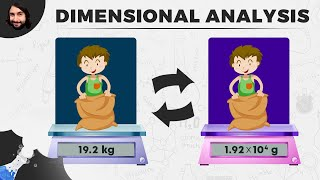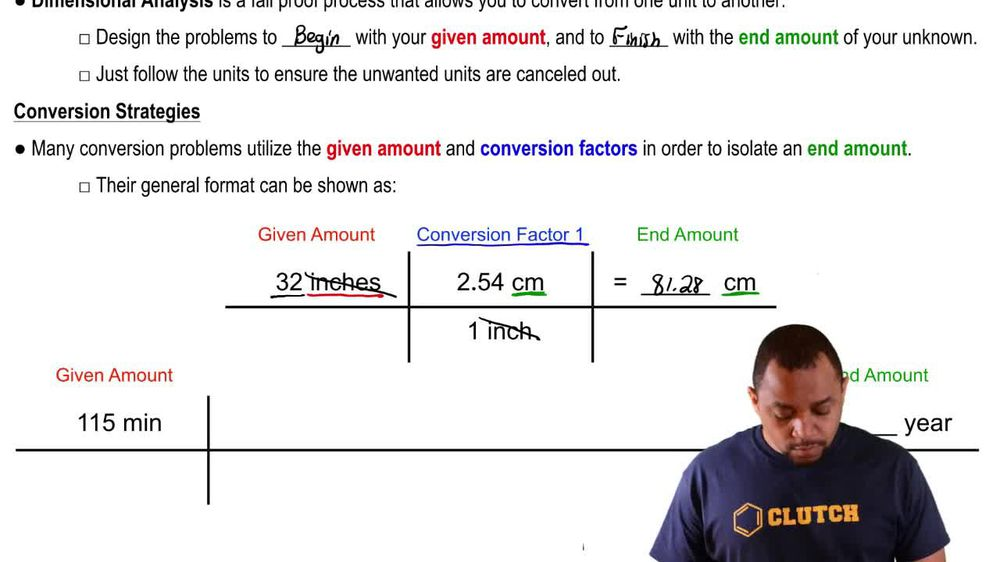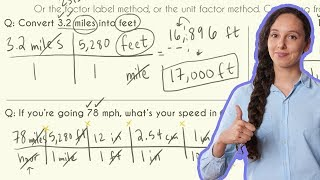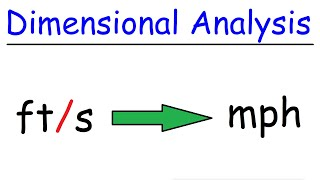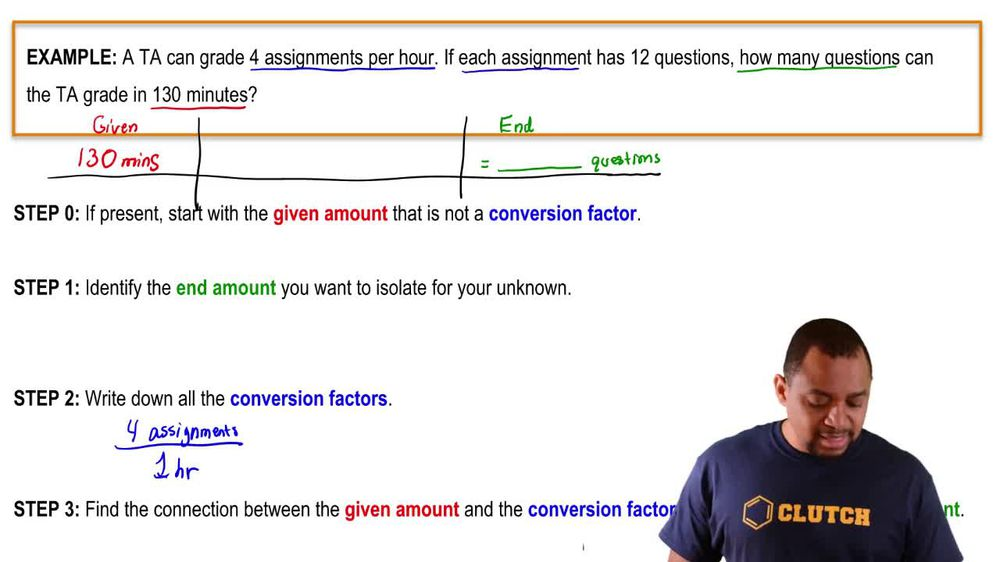Start typing, then use the up and down arrows to select an option from the list.
1. 1. Intro to General Chemistry2. Dimensional Analysis
Problem

# The height of a horse is usually measured in hands instead of in feet, where 1 hand equals 1/3 ft (exactly). (a) How tall in centimeters is a horse of 18.6 hands?

Relevant Solution1m
Play a video:
Hey, everyone were asked if we express a cat's height and finger measurements rather than feet where one ft equals 2.67 fingers, what is the height and inches of a 3.5 finger cat? Now, essentially our pathway here is going to be from our finger into our foot and lastly into inches. So using our dimensional analysis will start off with 3. fingers and we were told that we had 2. fingers per one fine. Using our conversion factors, we know that one ft contains 12 inches. So when we calculate this out and cancel out our units, we end up with a total of 16" and this will be our final answer. Now, I hope that made sense and let us know if you have any questions.